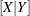# The GLM Procedure

### Absorption

Absorption is a computational technique used to reduce computing resource needs in certain cases. The classic use of absorption occurs when a blocking factor with a large number of levels is a term in the model.

For example, the statements

proc glm;
absorb herd;
class a b;
model y=a b a*b;
run;


are equivalent to

proc glm;
class herd a b;
model y=herd a b a*b;
run;


The exception to the previous statements is that the Type II, Type III, or Type IV SS for HERD are not computed when HERD is absorbed.

The algorithm for absorbing variables is similar to the one used by the NESTED procedure for computing a nested analysis of variance. As each new row of(corresponding to the nonabsorbed independent effects and the dependent variables) is constructed, it is adjusted for the absorbed effects in a Type I fashion. The efficiency of the absorption technique is due to the fact that this adjustment can be done in one pass of the data and without solving any linear equations, assuming that the data have been sorted by the absorbed variables.

Several effects can be absorbed at one time. For example, these statements

proc glm;
absorb herd cow;
class a b;
model y=a b a*b;
run;


are equivalent to

proc glm;
class herd cow a b;
model y=herd cow(herd) a b a*b;
run;


When you use absorption, the size of thematrix is a function only of the effects in the MODEL statement. The effects being absorbed do not contribute to the size of thematrix.

For the preceding example, a and b can be absorbed:

proc glm;
absorb a b;
class herd cow;
model y=herd cow(herd);
run;


Although the sources of variation in the results are listed as

a b(a) herd cow(herd)


all types of estimable functions for herd and cow(herd) are free of a, b, and a*b parameters.

To illustrate the savings in computing by using the ABSORB statement, PROC GLM is run on generated data with 1147 degrees of freedom in the model with the following statements.

data a;
do herd=1 to 40;
do cow=1 to 30;
do treatment=1 to 3;
do rep=1 to 2;
y = herd/5 + cow/10 + treatment + rannor(1);
output;
end;
end;
end;
end;
run;

proc glm data=a;
class herd cow treatment;
model y=herd cow(herd) treatment;
run;


This analysis would have required over 6 megabytes of memory for thematrix had PROC GLM solved it directly. However, in the following statements, the GLM procedure needs only amatrix for the intercept and treatment because the other effects are absorbed.

proc glm data=a;
absorb herd cow;
class treatment;
model y = treatment;
run;


These statements produce the results shown in Figure 45.17.

Figure 45.17: Absorption of Effects

The GLM Procedure

Class Level Information
Class Levels Values
treatment 3 1 2 3

 Number of Observations Read 7200 7200

The GLM Procedure

Dependent Variable: y

Source DF Sum of Squares Mean Square F Value Pr > F
Model 1201 49465.40242 41.18685 41.57 <.0001
Error 5998 5942.23647 0.99070
Corrected Total 7199 55407.63889

R-Square Coeff Var Root MSE y Mean
0.892754 13.04236 0.995341 7.631598

Source DF Type I SS Mean Square F Value Pr > F
herd 39 38549.18655 988.44068 997.72 <.0001
cow(herd) 1160 6320.18141 5.44843 5.50 <.0001
treatment 2 4596.03446 2298.01723 2319.58 <.0001

Source DF Type III SS Mean Square F Value Pr > F
treatment 2 4596.034455 2298.017228 2319.58 <.0001